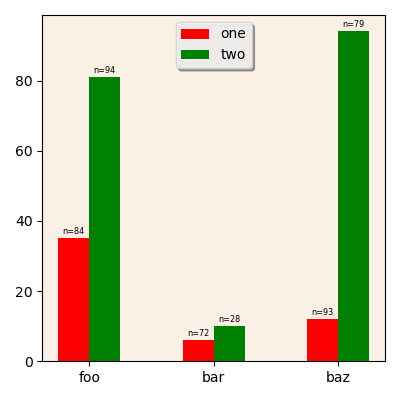# Drawing barplots¶

The `incenp.plotting.bar` module provides a `barplot` function intended to facilitate the creation of bar plots from multi-indexed data.

The module uses the same notion of tracks and subtracks as the `incenp.plotting.scatter` module.

## Sample data¶

Let’s create a multi-indexed DataFrame which we will use in the examples below:

```index = pd.MultiIndex.from_arrays([
['foo'] * 2 + ['bar'] * 2 + ['baz'] * 2 + ['qux'] * 2,
['one', 'two'] * 4
],
names=['first', 'second']
)
df = pd.DataFrame(np.random.randint(0, 100, size=(8,2)),
index = index, columns=['A', 'B'])
```

This creates a DataFrame with 2 columns (`A` and `B`) and 8 rows, indexed in two levels (level `first`, with 4 distinct values `foo`, `bar`, `baz`, and `qux`; and level `second`, with 2 distinct values `one` and `two`).

## Quick start¶

Here is a quick example illustrating the main points of the `barplot` function (`ax` is supposed to be a matplotlib.axes.Axes object):

```barplot(ax, df, column='A',
tracks=['foo', 'bar', 'bax'],
subtracks=['one', 'two'], subtrackname='second',
ncolumn='B')
ax.legend(['one', 'two'], loc='upper center')
```A sample bar plot.

The `column` parameter indicates which column in the DataFrame contains the value to plot. The `tracks` and `subtracks` parameters are used to select and distribute the rows along the tracks and subtracks, in a similar way to the `scatterplot` function of the `incenp.plotting.scatter` module.

The `ncolumn` parameter, if included, indicates which column in the DataFrame contains the number of samples, to be displayed on top of every bar.